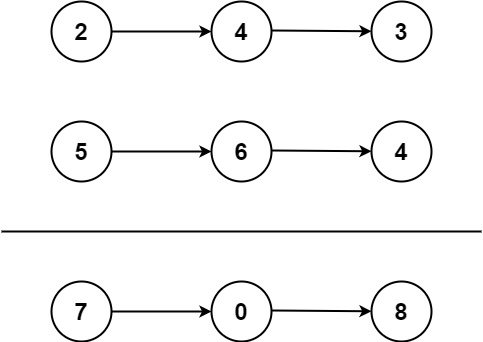Research

Problem Description

You are given two non-empty linked lists representing two non-negative integers. The digits are stored in reverse order, and each of their nodes contains a single digit. Add the two numbers and return the sum as a linked list.

You may assume the two numbers do not contain any leading zero, except the number 0 itself.

Example 1:Input: l1 = [2,4,3], l2 = [5,6,4]
Output: [7,0,8]
Explanation: 342 + 465 = 807.

Example 2:

Input: l1 = , l2 = 
Output: 

Example 3:

Input: l1 = [9,9,9,9,9,9,9], l2 = [9,9,9,9]
Output: [8,9,9,9,0,0,0,1]

Constraints:

• The number of nodes in each linked list is in the range [1, 100].
• 0 <= Node.val <= 9
• It is guaranteed that the list represents a number that does not have leading zeros.

Solution:

# class ListNode(object):
#     def __init__(self, val=0, next=None):
#         self.val = val
#         self.next = next
class Solution(object):
"""
:type l1: ListNode
:type l2: ListNode
:rtype: ListNode
"""

carry = 0
while l1 is not None and l2 is not None:
cur_digit = (carry + l1.val + l2.val) % 10
carry = (carry + l1.val + l2.val) / 10
cur_node.next = ListNode(cur_digit)
cur_node = cur_node.next

l1 = l1.next
l2 = l2.next

while l1 is not None:
cur_digit = (carry + l1.val) % 10
carry = (carry + l1.val) / 10
cur_node.next = ListNode(cur_digit)
cur_node = cur_node.next

l1 = l1.next

while l2 is not None:
cur_digit = (carry + l2.val) % 10
carry = (carry + l2.val) / 10
cur_node.next = ListNode(cur_digit)
cur_node = cur_node.next

l2 = l2.next

if carry != 0:
cur_node.next = ListNode(carry)
cur_node = cur_node.next

Before explaining the above solution, it should be prefaced that there is an another approach to this problem that isn’t explicitly forbidden, but at the same time would probably not be considered valid in an actual coding interview. That approach involves multiplying the digits of each number by powers of ten to obtain to integer primitives, adding them together, and then turning the result of that into a linked list for the solution. The above solution is, in fact, more efficient than this approach because it does not ever turn the linked lists into integers, but rather builds the result while traversing both lists at once.

On lines 15 and 16, the “result” data structure (the one that we are eventually going to return) is set up. head, the pointer to a ListNode structure that we are eventually going to return, is initialized as an empty node and an identical list node pointer to keep track of the current node is also initialized. This extra pointer is necessary because of how the linked list data structure works; when we are building the linked list, we are essentially traversing it and once we do so, we have no way of going back to the first element. Therefore, we use head to preserve the first (root) element of the linked list.

The numbers inside the linked lists are in reverse order, which at first sounds like an inconvenience, but it is in fact the way we do addition ourselves (at least on paper). Just as if we are doing the addition on paper, on lines 18 and 19, we compute the result of adding the digits of both numbers (plus the carry flag, which is initially zero) and then calculate the new carry flag (if the sum of the digits is 10 or more, then the integer division will yield 1 and otherwise it will be zero). We then set that value as the next element in our final result on line 20 and then traverse to it so that it is the current element. Note that we could not have set the result of the addition as the current element in the linked list, as the next pointer would be null and traversing to it would lose the reference to the list.

After advancing the next pointer of cur_node, we then have to advance the pointer in both of the input linked lists which represent the numbers to be added. This is analogous to moving on to the next pair of digits to add when doing addition by hand.

In the two while loops that follow, we are checking to see if one of the numbers has run out of digits (this means that one number has fewer digits than the other), and depending on which number is shorter, add the digits from the remaining number to our result in a similar manner as before until the longer number is exhausted.

Finally, when we are done with all of the digits from both numbers, we still need to check if there is a one to carry, and if there is then we need to add one more ListNode (containing a “1”) to the end of the result.

Previous Post

Next article